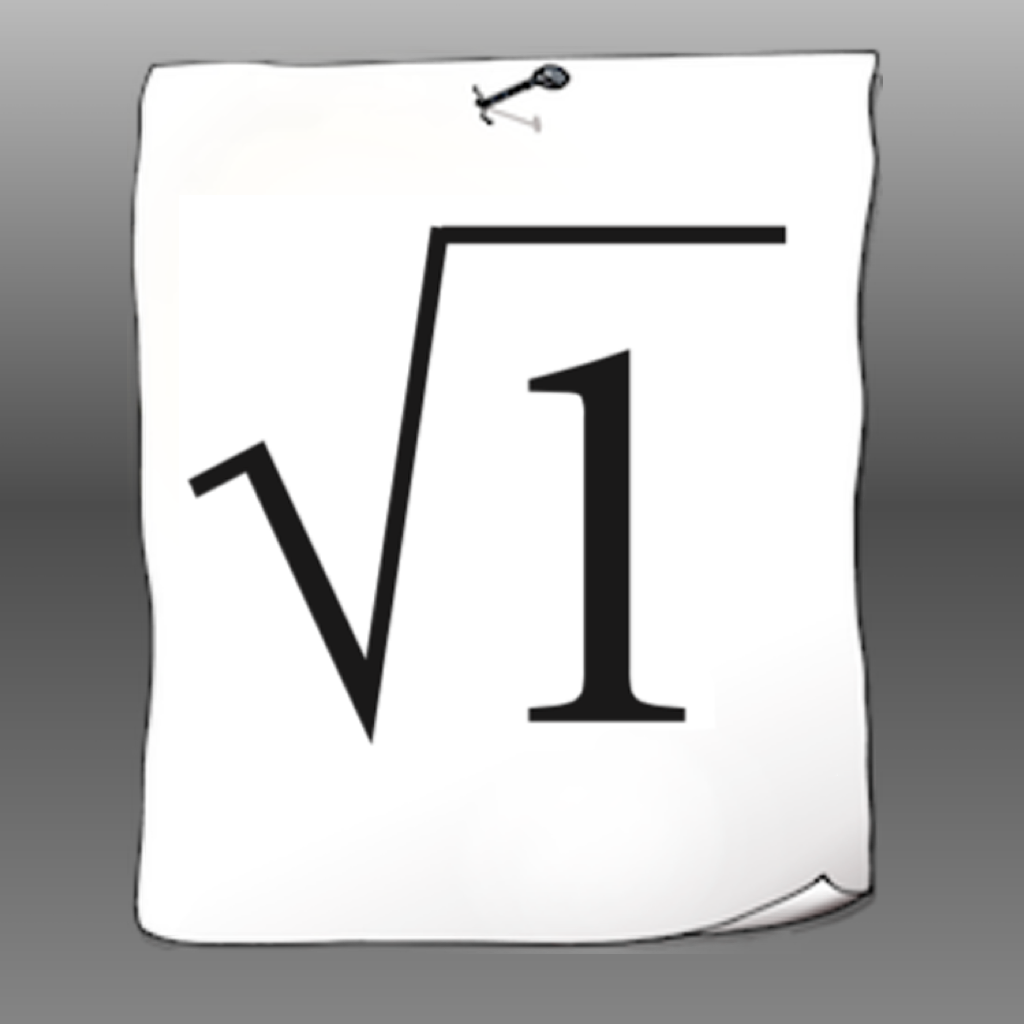en

# SpeedMaths!Practice your math skills in a fun and challenging way. The app is targeted to secondary school level (ages 13-15) and features an endless way to practice many of the basic mathematic skills needed.

The basic part of the app consists of different task were you need to provide the correct answer for 10 tasks within 75 seconds. When that is done you can continue with another 10 tasks and try to get as high streak as possible.

In the statistic and practice section you will be provided with results for each category to see within which category you need to practice more. You also have the possibility to practice a specific category before you give it another try.

The app contains endless tasks divided into those 30 categories:

Subtraction

Multiplication

Division

Priority rules

Multiplication by 10, 100 & 1000

Division by 10, 100 & 1000

Decimal form

Round to integer

Round to decimal

Decimal form - subtraction

Decimal form - multiplication

Decimal form - division

Sequence

Negative numbers - addition & subtraction

Negative numbers - multiplication & division

Exponents

Powers of ten

Square root

Length units

Weight units

Volume units

Area units

Volume units (cubic)

Average speed

Mean value

Median value

Mode value

Prime numbers